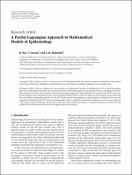## A partial Lagrangian approach to mathematical models of epidemiology.

 dc.contributor.author Naz, R. dc.contributor.author Naeem, I dc.contributor.author Mahomed, F.M. dc.date.accessioned 2016-06-06T14:00:49Z dc.date.available 2016-06-06T14:00:49Z dc.date.issued 2015 dc.description.abstract This paper analyzes the first integrals and exact solutions of mathematical models of epidemiology via the partial Lagrangian approach by replacing the three first-order nonlinear ordinary differential equations by an equivalent system containing one second order equation and a first-order equation. The partial Lagrangian approach is then utilized for the second-order ODE to construct the first integrals of the underlying system.We investigate the SIR and HIV models.We obtain two first integrals for the SIR model with and without demographic growth. For the HIV model without demography, five first integrals are established and two first integrals are deduced for the HIV model with demography. Then we utilize the derived first integrals to construct exact solutions to the models under investigation. The dynamic properties of these models are studied too. Numerical solutions are derived for SIR models by finite difference method and are compared with exact solutions. en_ZA dc.identifier.citation Naz, R., Naeem, I.,and Mahomed, F.M. 2015. A partial Lagrangian approach to mathematical models of epidemiology. Mathematical Problems in Engineering en_ZA dc.identifier.issn 1024-123X (Print) dc.identifier.issn 1563-5147 (Online) dc.identifier.uri http://hdl.handle.net/10539/20428 dc.language.iso en en_ZA dc.publisher Hindawi Publishing Corporation en_ZA dc.subject Diseases en_ZA dc.subject Finite difference method en_ZA dc.subject Nonlinear equations en_ZA dc.subject Numerical methods en_ZA dc.subject Ordinary differential equations en_ZA dc.subject Differential equations en_ZA dc.subject Population dynamics en_ZA dc.subject Population statistics en_ZA dc.title A partial Lagrangian approach to mathematical models of epidemiology. en_ZA dc.type Article en_ZA
##### Original bundle
Now showing 1 - 1 of 1Name:
A Partial Lagrangian Approach to Mathematical Models of Epidemiology.pdf
Size:
312.26 KB
Format: